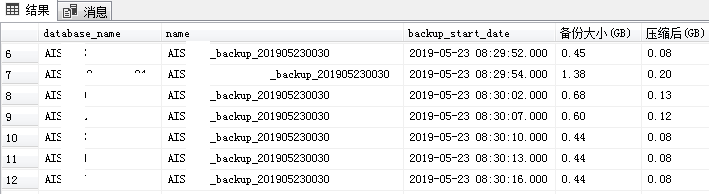## 小数乘法除法练习题批量生成

SQL SERVER 代码

–1.生成随机数
select top 5000
ceiling(rand(checksum(NEWID())) *1000) as x –生成3位以内随机整数
,ceiling(rand(checksum(NEWID())) *100) as y –生成2位以内随机整数
–cast(cast(rand(rand(number)100000000)10000000000000 as bigint)%1000 as dec(10,4)) as x –生成3位以内随机整数
–,cast(cast(rand(rand(number)100000000)1000000000000000 as bigint)%100 as dec(10,4)) as y –生成2位以内随机整数
–,number,rand(number)100000000,rand(rand(number)100000000)
,cast(0 as dec(28,6))as z
into #temp01
from master..spt_values a,master..spt_values b
where 1=1

–将整数转换为随机位小数
update a set
x=x/(case when cast(rand(x)100000000 as int)%4=1 then 1000 when cast(rand(x)100000000 as int)%4=2 then 100 when cast(rand(x)100000000 as int)%4=3 then 10 else 1 end), –将3位整数随机转换为小数 y=y/(case when cast(rand(y)100000000 as int)%3=1 then 100 when cast(rand(y)*100000000 as int)%3=2 then 10 else 1 end )–将2位整数随机转换为小数
from #temp01 a

–2.清理数据
update #temp01 set z=x*y –计算乘积得数

–去除得数为0的计算
delete from #temp01 where z=0

–去除全整数计算
delete from #temp01 where (x-cast(x as int)=0 and y-cast(y as int)=0)

–去除两个乘数都是小数的计算
delete from #temp01 where cast(x as int)=0 and cast(y as int)=0

–去除有一个乘数是1的计算
delete from #temp01 where x=1 or y=1

–3.输出结果
–select * from #temp01
–输出小数乘法题
select cast(x as float)as 乘数,’x’ 乘,cast(y as float)as 乘数,’=’as 等于,cast(z as float)as 积 from #temp01 order by NEWID()
–输出小数除法题
select cast(z as float)as 被除数,’÷’ 除,cast(y as float)as 除数,’=’as 等于,cast(x as float)as 商 from #temp01 order by NEWID()

27.9 x 0.8 = 22.32
33.6 x 9.2 = 309.12
0.18 x 14 = 2.52
0.425 x 4.2 = 1.785

3312.4 ÷ 5.2 = 637
72.416 ÷ 7.3 = 9.92
7.5287 ÷ 0.79 = 9.53
37.037 ÷ 77 = 0.481

5000道题目下载： 小数计算题一万道

## SQL Server 备份检查及监控## WebApi+SQLServer定时任务方案

1.首先是写一个可以http调用的api程序。以下程序调用路径是 /api.aspx?act=GO

2. 在SQLServer维护计划里添加T-SQL语句任务，并设置按周期执行。

3. 以上SQL里用到的函数组件

P_Url_SendRequest  连接URL，支持POST\GET 支持返回值

MSSQL访问WebServer接口代码 – P_Url_SendRequest

parsejson JSON解析函数

MSSQL JSON函数-parseJSON

P_Send_TextMsg 企业微信消息推送执行结果

MSSQL调用企业微信发送消息接口封装-P_Send_TextMsg

## SQL Server 防注入参数化之 Where in

where in 字符串：

字符串转换参数(int类型)table函数如下：

## SVN 自动化部署及强制注释

1.设置提交时必须填写说明

`findstr "........" 匹配8个英文字符或4个中文字符。`

2.按提交的注释内容，自动更新部署到服务器制定目录。

pre-commit钩子中是临时版本号，`%SVNLOOK% log %REPOS% -t %REV%`